Scalar Potentials: Their Gradient Fields and Visualization

Setting up the combined potential due to three point potentials as an example to visualize gradients, divergences, and curls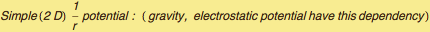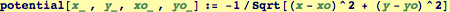A field source located a distance 1 south of the origin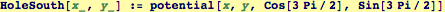Sources located distance 1 at 30° and 150°: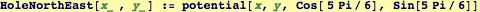Function that returns the two dimensional (x,y) gradient field of any function declared a function of two arguments: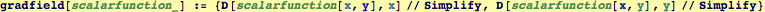Generalizing the function to any arguments: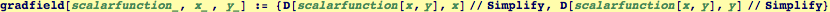The sum of three potentials: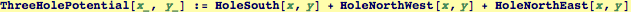f(x,y) visualization of the scalar potential: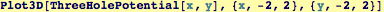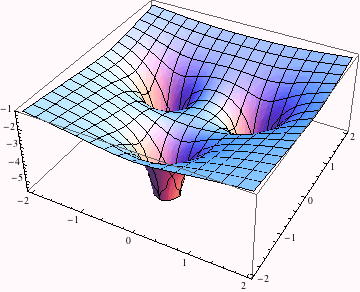Contour visualization of the three-hole potential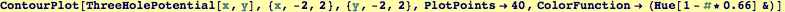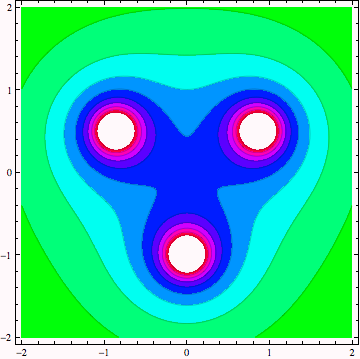Created by Wolfram Mathematica 6.0  (21 September 2007)